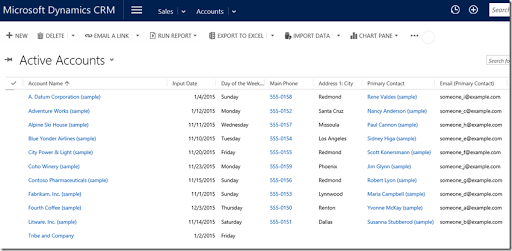## Monday, November 30, 2015

### Calculating the Day of the Week Without Code

This blog is inspired by a forum post I read where someone asked to display the day of the week in a CRM view. Unfortunately, I cannot find the request now but it is possible (and without code).In the above, the Input Date is the date we want the date for and the Day of the Week shows the correct day.

### The Calculation

For this one we need the following fields.Working backwards:

• Day of the Week: An option set of the days of the week (set via a workflow)
• DOTW Number: A Whole Number which shows a number representing the correct day of the week
(a calculated field and, in this case, a number between –3 and 3)
• Dodgy DIV: Strictly speaking, not a mathematical DIV but close enough for our purposes. In our case, this represents the week our Input Date is in. It is a calculated field.
• Input Date: The date of interest, entered by the user.
• Reference Date: A date we know the day of the week for. In this case, 1/1/2015 which was a Thursday

To anyone outside of the USA, Belize, or the Federated States of Micronesia, I apologize for using “Middle Endian” formatting for my dates. It is a blatant play to the American market on my behalf and does not affect the calculations.

In terms of the Reference Date, my preference was to incorporate this into the Dodgy DIV calculation but I could not figure out how to input a fixed date e.g. “01/01/2015” into the calculated field. CRM either thought it was an integer or thought it was a string. If anyone knows how to do this, please leave a comment at the end.

Given I could not feed my reference date directly into the Dodgy DIV calculation, I had to automatically enter it into a field via a Business Rule. Here it is.I tried using the Set Default Value action but struggled to get it to behave so I just used the Set Value action in the end. I also was forced to put in a condition so I selected one which is always true.

With this value set, I could then calculate the Dodgy DIV.A couple of issues with this formula, which is why it is dodgy. What I wanted it to do was calculate the difference in days between 1/1/2015 and our Input Date and then divide it by seven. It turns out that the DiffInDays function has a pretty huge bug in it, in that it gets the difference in days wrong. I found that if I put in the same two dates into the DiffInDays formula, it returned –1 and not 0, as expected. This is why I add one.

The second issue is, in C (the language I used to code in a loong time ago), if you divided two numbers and put them into an integer variable, it rounded down, effectively being a DIV operation. Dynamics CRM does not play this way and applies a normal rounding operation (x.5 or more goes up and less than x.5 goes down).

Using my Dodgy DIV, I can now calculate the day of the week. The DOTW Number (Day of the Week Number) is also a calculated field.This is where the clever trick comes in (if I do say so myself). Here we calculate the difference in days again, add one to account for the bug in the DiffInDays formula and take away the Dodgy DIV value, multiplied by 7. Because the Dodgy DIV field has applied a rounding, the difference is the Modulus (also know as the remainder) or it would be for a pure DIV function. In our case we generate a number between –3 and 3, rather than a number between 0 and 6.

Finally, we have our days of the week. Unfortunately there is no simple way to set the Option Set Value with a formula using a number so I had to use a real time workflow.The workflow is triggered on creation of the record and on the changing of the Input Date field. There are seven IF statements, one for each day of the week, linking the DOTW Number to the right day.

Once all of this is done it works like a treat, with the day value being set on the saving of the record. It is then a case of adding the Day of the Week field to the view you want to see it in.### Conclusions

I like this solution as it opens the way for quite a few other requests seen in CRM systems. For example, with the day of the week we can check whether a day is a work day when setting an appointment. Although I have not fully figured it out how yet, I imagine we could also use a similar technique to work out the number of working days between two dates. So many possibilities thanks to calculated fields.

If you are not exploring how calculated fields can help you manage your business processes in Dynamics CRM, perhaps you should because, as you can see above, they open up a wealth of options, previously inaccessible, without using code.